The core math topics covered in grade 5 typically are:

Numbers and Operations

• multiplying and dividing by powers of ten
• multiplying and dividing large whole numbers in columns
• rectangular arrays
• add and subtract fractions with unlike denominators
• multiply fractions by fractions and / or whole numbers
• divide fractions by whole numbers
• reading, writing and comparing decimals
• add, subtract, multiply and divide decimals
• use of parenthesis in expressions
• word problems involving fractions and decimals
• exponents and powers of ten

Geometry

• rectangular prisms
• volumes of 3 dimensional shapes
• coordinate planes
• categorizing 2D shapes by various attributes

Measurement

• convert measurements between different types of units (metric and customary)
• multi-step word problems in measurement

Data Analysis

• create line plots to display data
• solve real world word problems by referring to line plots

The common core math standards for grade 5 are also worth reviewing.

## Math worksheets for grade 5

Choose from hundreds of free grade 5 math worksheets organized by topic:

### Fractions

Place Value & Rounding Add & Subtract Fractions
Add & Subtract Multiply & Divide Fractions
Multiply & Divide Converting Fractions
Order of Operations

### Decimals

Measurement Fractions to / from Decimals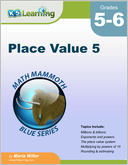Place Value 5 Numbers up to the trillions, rounding huge numbers and using a calculator.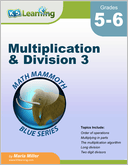Multiplication and Division 3 Multiplication and division topics including order of operations, multiplying in parts, long division, divisibility rules, factors and prime factors, greatest common factor, least common multiple and ratios.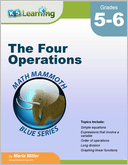The Four Operations Topics related to the four operations and algebra including balance problems and equations, expressions involving a variable, order of operations, the multiplication algorithm, long division, powers and exponents and the coordinate grid.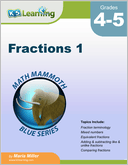Fractions 1 Focus on the addition and subtraction of fractions with like and unlike denominators.  Also mixed numbers, equivalent fractions and comparing fractions.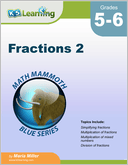Fractions 2 Simplifying fractions and the multiplication and division of fractions and mixed numbers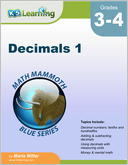Decimals 1 Introduction to decimals (tenths and hundredths). Adding and subtracting decimals mentally and in columns.  Decimals and money.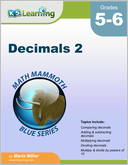Decimals 2 Adding, subtracting, multiplying and dividing decimals.  Multiplying and dividing by powers of 10. Rounding and estimating.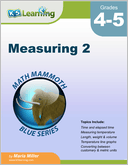Measuring 2 Length, weight, capacity, temperature and time.  Focus on conversion between different measuring units.  Customary and metric units used.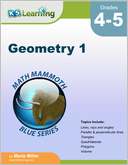Geometry 1 Overview focused on basic plane geometry. Topics include lines, rays and angles; measuring angles; parallel and perpendicular lines, shapes, area, volume. Also rectangular prisms and surface area.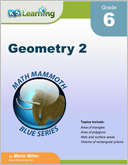Geometry 2 Classifying triangles and quadrilaterals, area of common polygons, circumference and area of circles, conversion between units of area.  Nets, surface area and volumes of common solids.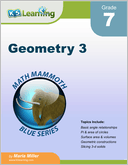Geometry 3 Angles, classifying triangles, congruent and similar figures, transformations, ruler and compass, square roots and the Pythagorean Theorem.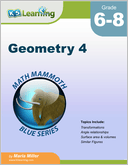Geometry 4 Geometric transformations and congruence; translations, reflections, rotations, sequences of transformations. Similar figures, corresponding angles, angle relationships and parallel lines. Volume of prisms, cylinders, pyramids, cones and spheres.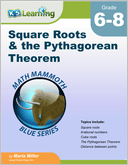Square Roots & the Pythagorean Theorem Square roots, the Pythagorean Theorem, applications of the Pythagorean Theorem and a proof of the Pythagorean Theorem.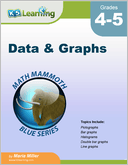Data and Graphs Statistics and common graphs and related topics including pictographs, bar graphs, histograms, double bar graphs, multiple line graphs, circle graphs and mean / median / mode.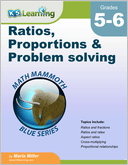Ratios, Proportions & Problem Solving Ratios and fractions, ratios and rates, cross multiplying, proportional relationships, aspect ratios, scaling figures, bar models and significant digits.